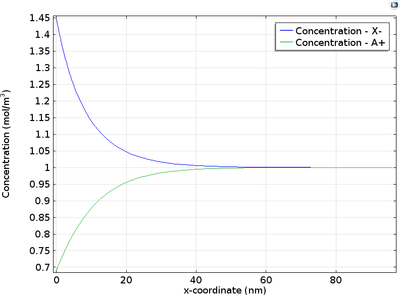# アプリケーションギャラリ

## Diffuse Double Layer

Application ID: 21981

At the electrode-electrolyte interface, there is a thin layer of space charge in a diffuse double layer. This may be of interest when modeling devices such as electrochemical capacitors and nanoelectrodes.

This tutorial example shows how to couple the Nernst-Planck equations to the Poisson equation, in order to describe diffuse double layer according to a Gouy-Chapman-Stern model. The physics interfaces Electrostatics and Transport of Diluted Species are coupled under conditions of:

• zero current
• cell size much greater than Debye lengthThis model example illustrates applications of this type that would nominally be built using the following products: